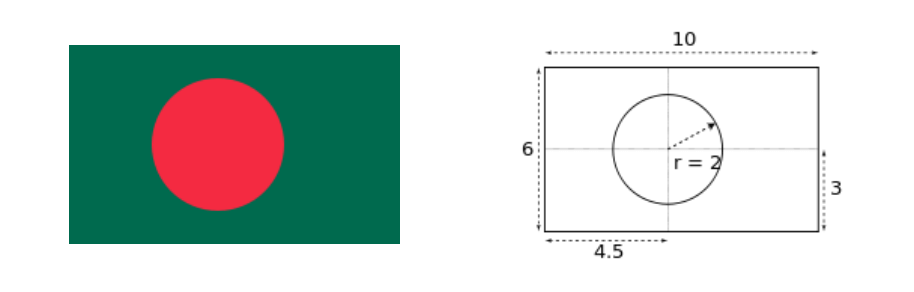Problems
229 - The national flag of Bangladesh (II)

The national flag of Bangladesh (II)

Time Limit: 1 sec

The Problem

The national flag of Bangladesh is bottle green in color and rectangular in size with the length (L) to width(W) ratio of 10:6. It bears a red circle on the background of green. It maintains the length (L) to radius (R) ratio of 5:1. If the lenght is can you find the width (W) and radius (R).

The color in the background represents the greenery of Bangladesh while the red circle symbolizes the rising sun and the sacrifice of lives in our freedom fight.

Example:

If the length(L) is 10 then width(W) should be 6 and radius(R) should be 2.The Input

The input file contains only one integer numbers L , which is the length of the flag (L<=1500).

The Output

Output will show the two integer numbesr which are the width(W) and radius(R) of the flag seperated by space in a separate line.

Sample Input

10

Sample Output

6 2

Problem Setter: Shahin ShamS